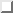Data Science Desktop Survival Guide by Graham WilliamsDesktop Survival Project Home Preface Data Science Introducing R R Constructs R Tasks R Strings R Read, Write, and Create Data Template Data Exploration Data Wrangling Data Visualisation Statistics ML Template ML Scenarios ML Activities ML Applications ML Algorithms Cluster Analysis Decision Trees Computer Vision Graph Data Privacy Literate Data Science Coding with Style Resources Bibliography Index

## Chapter: Decision Trees

20200815 Decision trees are widely used in data mining and well supported in R (R Core Team, 2020). Decision tree learning deploys a divide and conquer approach, known as recursive partitioning. It is usually implemented as a greedy search using information gain or the Gini index to select the best input variable on which to partition the dataset at each step.

Here we introduce rattle and rpart for building decision trees. We begin with a step-by-step example of building a decision tree using Rattle, and then illustrate the process using R begining with Section. We cover both classification trees and regression trees.

We have briefly introduced decision trees as an algorithm in Sectionand Chapter 12 uses decision trees as the model builder to demonstrate the model template. Examples of decision tree induction are available through the rain, iris, and pyiris packages from MLHub.

Subsections